# ER Diagrams to Tables | Practice Problems

## ER Diagrams to Tables-

Before you go through this article, make sure that you have gone through the previous article on ER Diagrams to Tables.

After designing an ER Diagram,

• ER diagram is converted into the tables in relational model.
• This is because relational models can be easily implemented by RDBMS like MySQL , Oracle etc.
• The rules used for converting an ER diagram into the tables are already discussed.

In this article, we will discuss practice problems based on converting ER Diagrams to Tables.

## Problem-01:

Find the minimum number of tables required for the following ER diagram in relational model-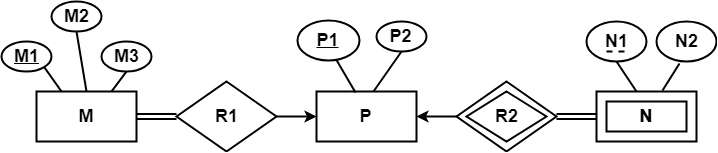## Solution-

Applying the rules, minimum 3 tables will be required-

• MR1 (M1 , M2 , M3 , P1)
• P (P1 , P2)
• NR2 (P1 , N1 , N2)

## Problem-02:

Find the minimum number of tables required to represent the given ER diagram in relational model-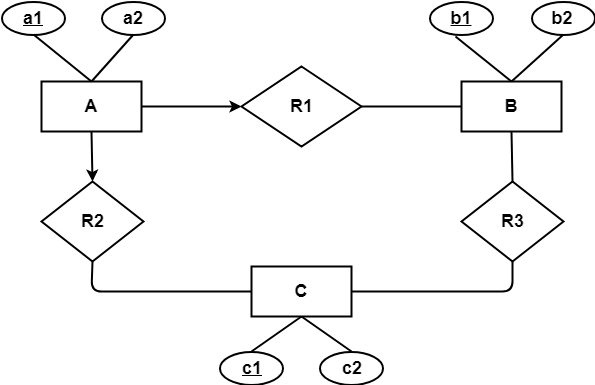## Solution-

Applying the rules, minimum 4 tables will be required-

• AR1R2 (a1 , a2 , b1 , c1)
• B (b1 , b2)
• C (c1 , c2)
• R3 (b1 , c1)

## Problem-03:

Find the minimum number of tables required to represent the given ER diagram in relational model-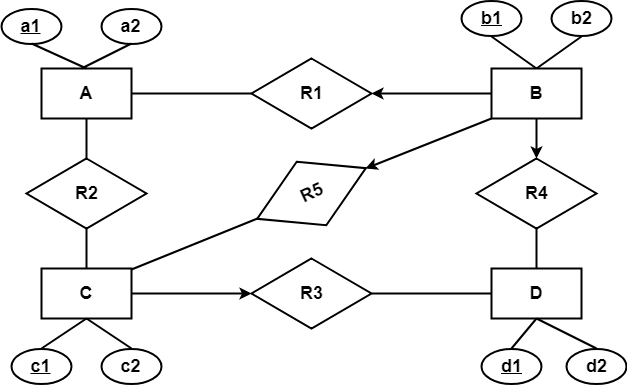## Solution-

Applying the rules, minimum 5 tables will be required-

• BR1R4R5 (b1 , b2 , a1 , c1 , d1)
• A (a1 , a2)
• R2 (a1 , c1)
• CR3 (c1 , c2 , d1)
• D (d1 , d2)

## Problem-04:

Find the minimum number of tables required to represent the given ER diagram in relational model-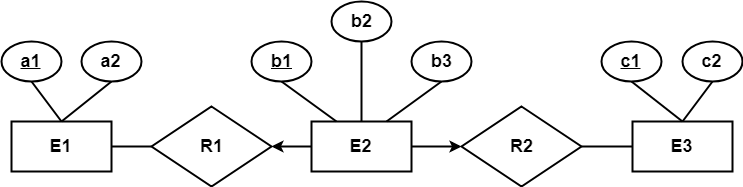## Solution-

Applying the rules, minimum 3 tables will be required-

• E1 (a1 , a2)
• E2R1R2 (b1 , b2 , a1 , c1 , b3)
• E3 (c1 , c2)

## Problem-05:

Find the minimum number of tables required to represent the given ER diagram in relational model-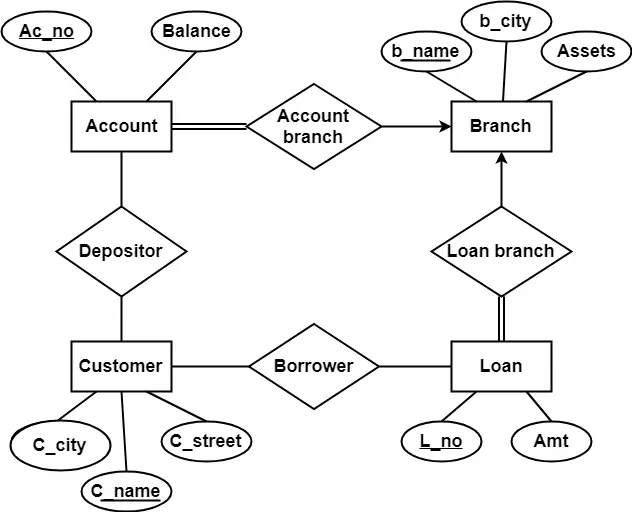## Solution-

Applying the rules that we have learnt, minimum 6 tables will be required-

• Account (Ac_no , Balance , b_name)
• Branch (b_name , b_city , Assets)
• Loan (L_no , Amt , b_name)
• Borrower (C_name , L_no)
• Customer (C_name , C_street , C_city)
• Depositor (C_name , Ac_no)

Next Article- Constraints in DBMS

Get more notes and other study material of Database Management System (DBMS).

Watch video lectures by visiting our YouTube channel LearnVidFun.

SummaryArticle Name
ER Diagrams to Tables | Practice Problems
Description
Practice problems based on converting ER diagram to tables. ER diagram is converted into the tables so that it can be easily implemented by any Relational Database Management System. We use the following rules for converting ER diagrams to tables.
Author
Publisher Name
Gate Vidyalay
Publisher Logo